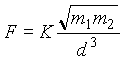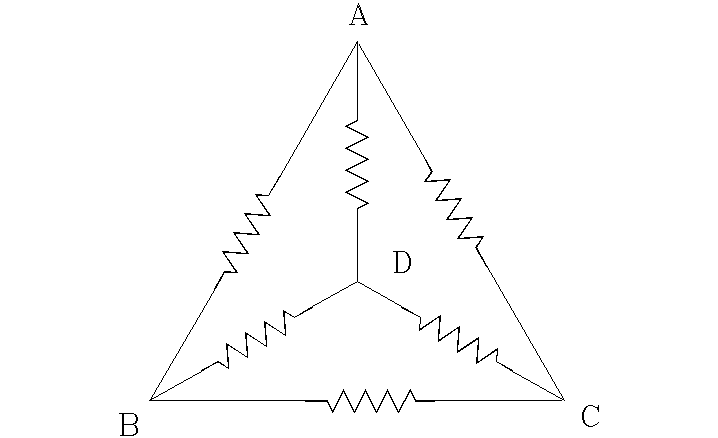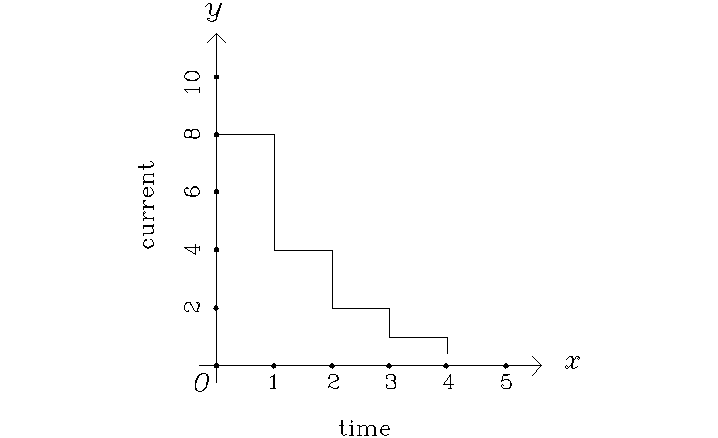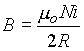SCHOLASTIC APTITUDE TEST 2000

PHYSICS

 Time: ONE Hour Max. Marks: 60

• Answers must be written either in English or the medium of instruction of the candidate in high school.
• Answer all the questions in the booklets provided for the purpose.
• There will be no negative marking.
• The relevant working in arriving at an answer has to be shown wherever required. However if the answer can be arrived at without detailed working, write only the answer
• Use of calculators or graph papers is not permitted.
• All questions carry equal marks

1. A body floats with half its volume submerged in a liquid A. The same body floats with three fourth of its volume submerged in a liquid B. Equal masses of these two liquids are mixed to form a homogeneous mixture and the body is floated in this mixture again. The fraction of the volume of the body that would be seen above the surface of the mixture is                          .
2. A line of cyclists separated by 50 m are travelling along a road at a constant speed of 4 m/s. Another cyclist is riding his bicycle at a constant speed of 6 m/s. The time taken by him to cross 10 cyclists is                                    if he is travelling in the same direction as the others and                            if he is travelling in a direction opposite the others.
3. A particle starting from rest accelerates at 2 m/s2 for some time and then travels uniformly and passes a point 175 m from the starting point 20 s after start. The velocities of the particle at the moment of passing the 20th m and 30th m marks are               and             .
4. A body is dropped from a certain height. 2 seconds later another body is projected vertically upward from the same point at 20 m/s. The distance between the first and second bodies 5 seconds after the projection of the second body is                                  . ( acceleration due to gravity = 10 ms–2 )
5. A boy drops stones from the roof of a building on to the ground 9 m below. The first stone released by him strikes the ground at the moment he has just released the fifth stone. The second, third and fourth stones are at heights of                      ,                         and                       above the ground at this moment. ( acceleration due to gravity = 10 ms–2 )
6. A pendulum has a time period of 2 s at a place where acceleration due to gravity is 9.83 m/s2. To have the same time period of 2 seconds at a place where acceleration due to gravity is 9.78 m/s2, it's length must be                                     .
7. A beaker with negligible water equivalent contains some water of mass m. Some mass of ice at 0° c is added to this water. The mass of ice added being equal to half the mass of water in the beaker and all the ice added just melts. If half of this mass of ice were to be added, the final temperature would have been                                .
8. Suppose gravitational force between two masses were to be given bywhere k is some constant. Two equal masses attract each other with a certain force when the distance is d. If each of the masses is doubled, the distance between them must be increased                   times for the force to remain the same as earlier.
9. If end correction is neglected it is possible to obtain resonance for lengths of air column which are in the ratio 1:3:5:7: ………….. ( This ratio is an approximation ). A meter long tube with its lower end closed is held vertically. A vibrating tuning fork of frequency 480 Hz is held at the mouth of the tube and water is slowly poured in to the tube. Shortest and longest lengths of the water column for which resonance is obtained are                   and                    . ( Velocity of sound in air is 345.6 m/s)
10. A candle flame and a screen are 180 cm apart. A lens is placed between them. For a certain position of the lens a twice magnified clear image is obtained on the screen. The lens is now moved away from the candle through 30 cm. To obtain another clear image on the screen, the distance through which the screen has to be moved is                          and the new magnification of the image is                                .
11. A ray of light incident normally on one face of an equiangular prism, gets internally reflected from the second face and emerges through the base of the prism. The angle of deviation of the ray is                         . ( Draw a neat ray diagram. )
12. 110 Particles are chasing each other on a circular path of radius 7 m. The speed of any particle is 2 m/s. Number of particles crossing any radial line every second is                    . If each of the particles carries a charge of 4 microColoumb, the average current in the path is                          .
13. All the resistances shown are equal. With resistance between A and D removed and the resistance between different points is measured. If the resistance between points B and C is R, the resistance between points A and B would be14. A potential difference applied to the ends of a wire causes a certain current to flow through it and a certain amount of heat to develop in it. The wire is stretched to obtain a new thin wire of half the original thickness. If the same potential difference is applied to the ends of the new wire, the heat developed _______________ times and the current flowing __________ times.
15. The current in a wire of resistance 10 Ohm varies as shown. The current remains constant for one second and in the next second it is half of the value in the previous second. If the initial value of the current is 8 A, the heat produced in the wire in 5 seconds is                            joule, and the charge that flows across any section of the wire in this time is                            .16. A house uses 5 lamps of 40 W each for 5 hours a day and an electrical heater of 1000 W for 1 hour a day. The energy consumed in a month of 30 days in kWh is                        . The mass ( in milligram ) that is equivalent to this energy is                            .
17. Magnetic field produced by a circular coil of radius R and having N turns carrying a current 'i'is given by. The magnetic field is perpendicular to the plane of the coil and is directed out of the plane if the current is anticlockwise. Two concentric coils made of wires of same length 'L' are made in to coils of radii R and 2R and have same current 'i' flowing through them in the same sense and are in the same plane. The magnetic field at the center of the coils is                            . (Permeability of free space m o=  4p  x 10–7 Hm–1)
18. An electron starting from rest is accelerated through a potential difference of 182 V. The kinetic energy (in joule ) gained by it is                            and it's velocity is                     . ( mass of electron m = 9.1 x 10-31 kg, charge of electron e = 1.6 x 10-19 C ).
19. A charged particle moving south west at right angles to a magnetic field experiences a force vertically upwards. This charged particle would not experience any force if it moved in a direction                       .
20. B and C are constituents of a nucleus A. The binding energy of nucleus A having three nucleons of type B and four nucleons of type C in MeV is                      . (Mass of B = 1.00733 amu, Mass of C = 1.00867 amu and Mass of A = 7.01586 amu)